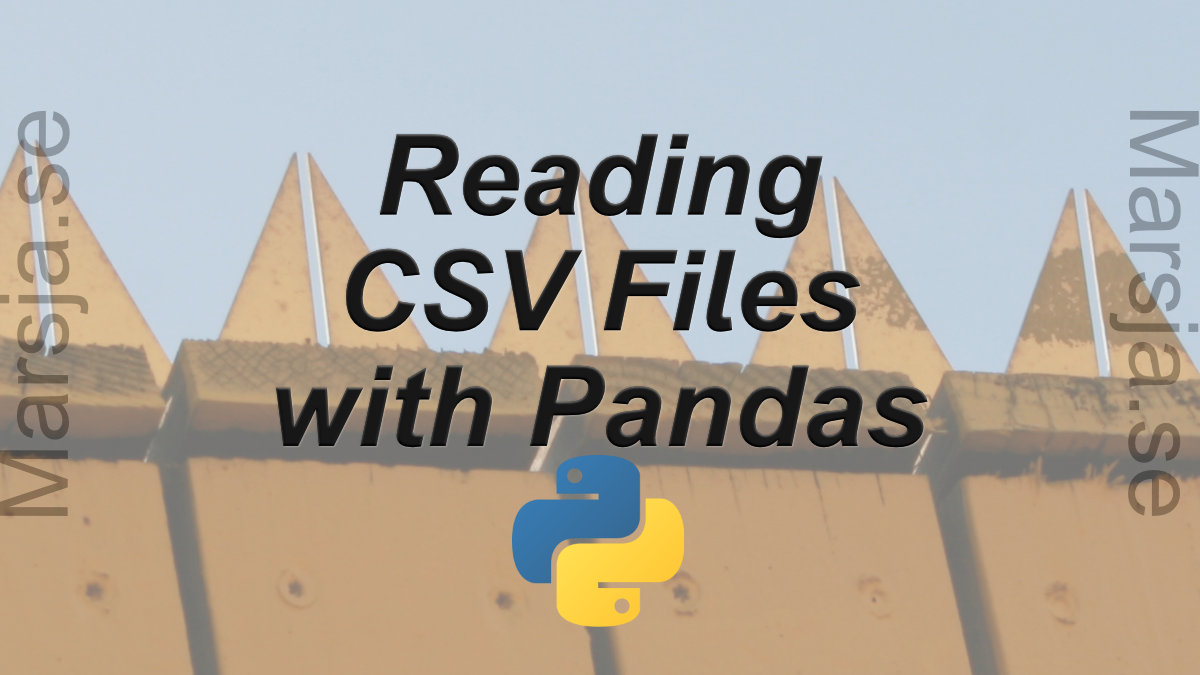In the first section, we will go through, with examples, how to read a CSV file, how to read specific columns from a CSV, how to read multiple CSV files and combine them to one dataframe, and, finally, how to convert data according to specific datatypes (e.g., using...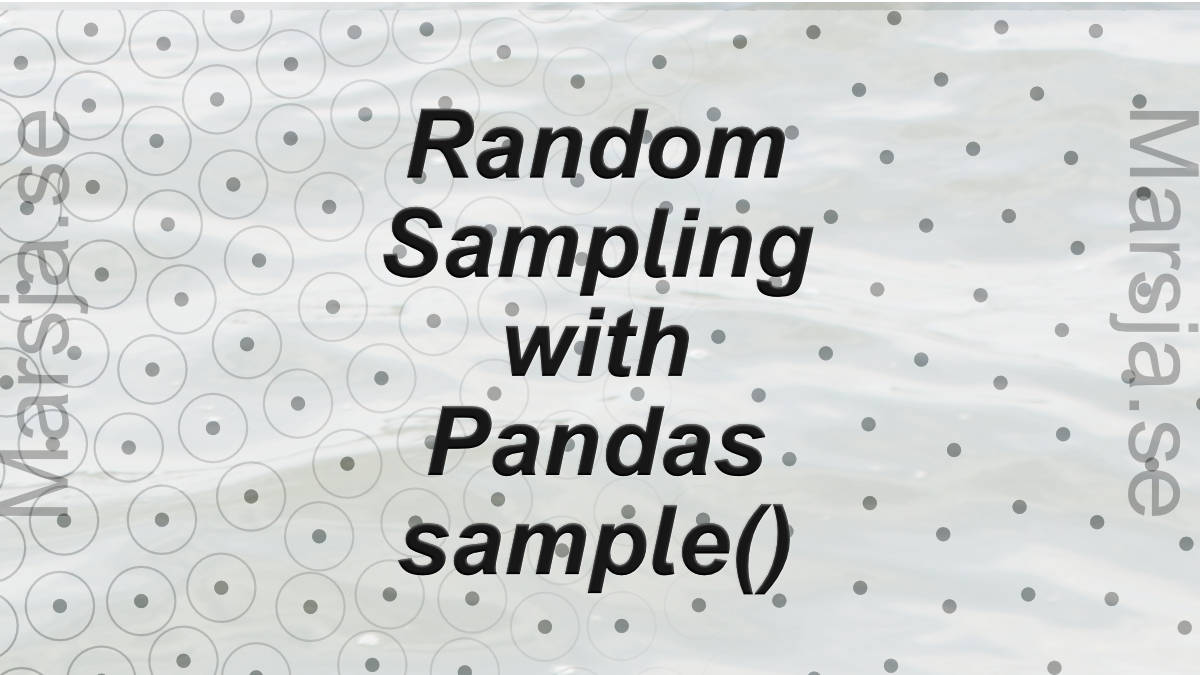## How to use Pandas Sample to Select Rows and Columns

In this tutorial, we will learn how to use Pandas sample to randomly select rows and columns from a Pandas dataframe. There are some reasons for randomly sample our data; for instance, we may have a very large dataset and want to build our models on a smaller sample...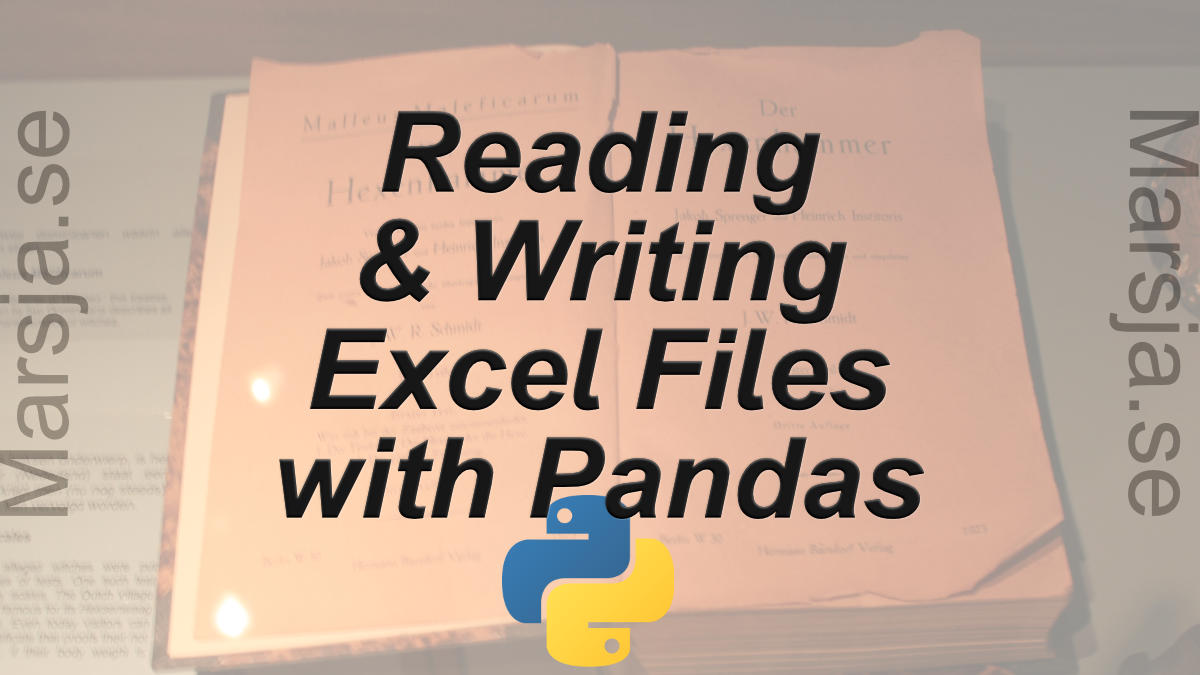## Pandas Excel Tutorial: How to Read and Write Excel files

In this Pandas Excel tutorial, we will learn how to work with Excel files in Python. It will provide an overview of how to use Pandas to load xlsx files and write spreadsheets to Excel. In the first section, we will go through, with examples, how to use Pandas...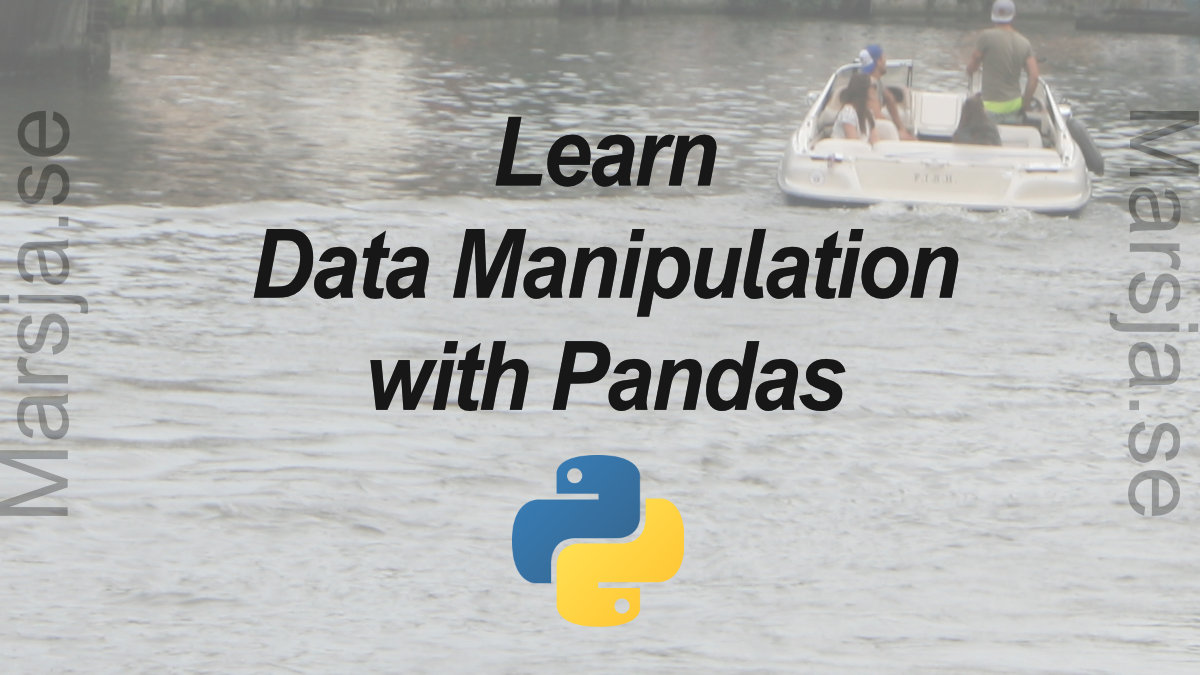## Data Manipulation with Pandas: A Brief Tutorial

Learn three data manipulation techniques with Pandas in this guest post by Harish Garg, a software developer and data analyst, and the author of Mastering Exploratory Analysis with pandas. Modifying a Pandas DataFrame Using the inplace Parameter In this section,...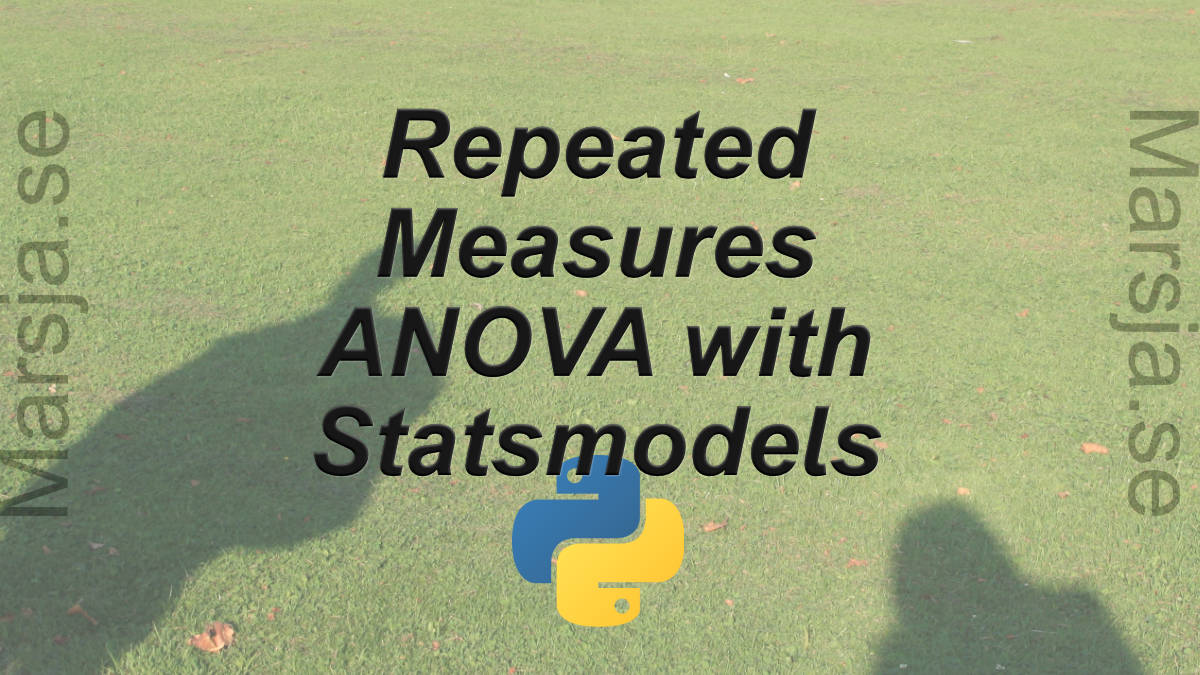## Repeated Measures ANOVA in Python using Statsmodels

In this brief Python data analysis tutorial we will learn how to carry out a repeated measures ANOVA using Statsmodels. More specifically, we will learn how to use the AnovaRM class from statsmodels anova module. To follow this guide you will need to have Python,...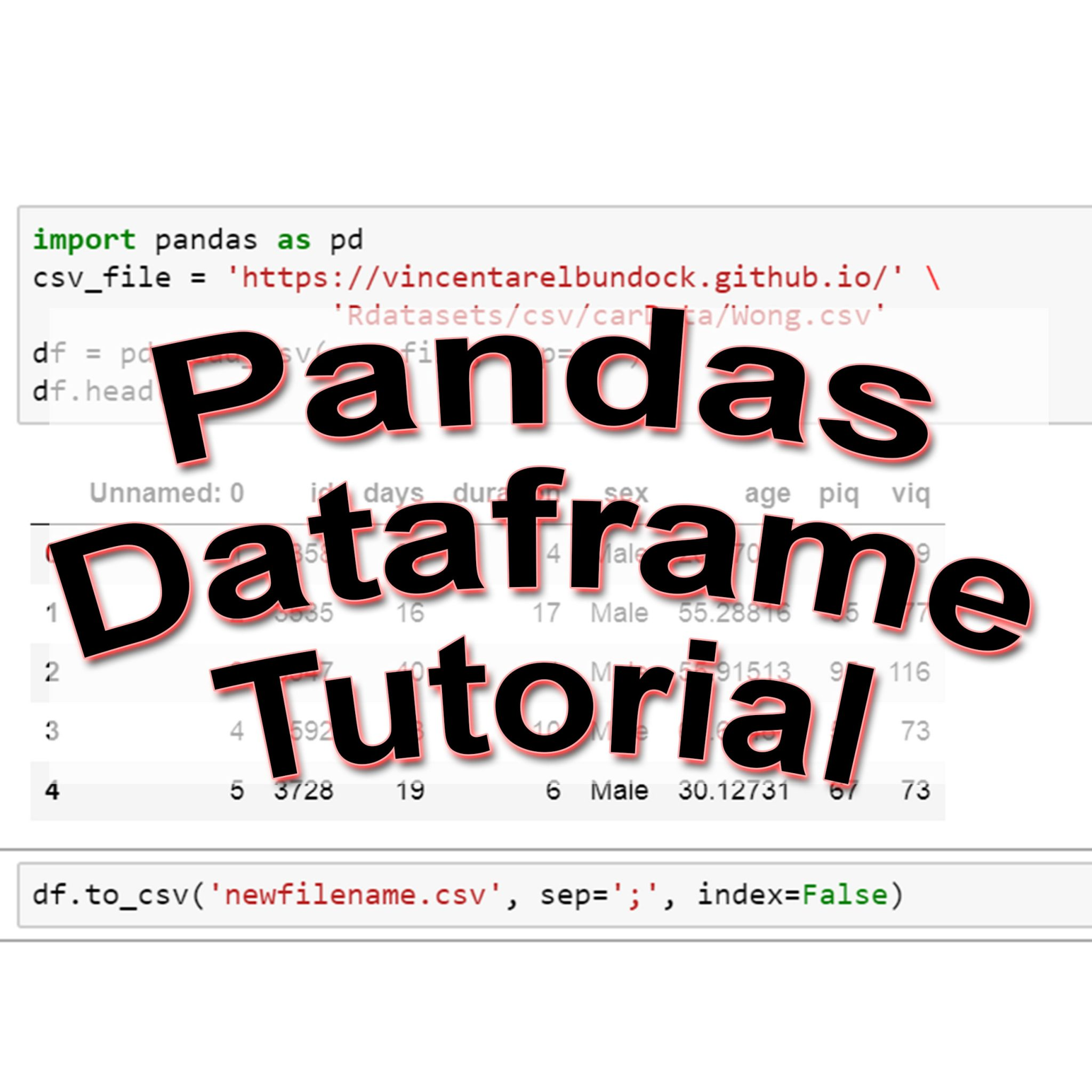## A Basic Pandas Dataframe Tutorial for Beginners

In this Pandas tutorial, we will learn how to work with Pandas dataframes. More specifically, we will learn how to read and write Excel (i.e., xlsx) and CSV files using Pandas. We will also learn how to add a column to Pandas dataframe object, and how to remove a...